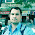Capacitance of a 3ph Line With Equilateral Spacing

Consider the three conductors a, b and c of 3 phase overhead transmission line having the charges qa , qb and q respectively as shown in Fig. 1. Let the conductors be separated from each other by a distance of d from each other and placed on the vertices of equilateral triangle.F ig. 1
The radius of each conductor is say r. The voltage Vab of the three phase line due to only charges on conductors a and b is given by,
Voltage Vab due to only charge q is zero as uniform charge distribution over the surface of the conductor is equivalent to a concentrated charge at the centre of conductor.
Considering all the three charges in writing the voltage equation we have,
Adding equations (1) and (2),
The voltages are sinusoidal and expressed as the phasors. In absence of other charges in the vicinity the sum of the charges is zero i.e.
q + q +  q = 0
...            q +  qc  = - qa
The Fig. 2 shows phasor diagram of balanced voltages of three phase line.Fig. 2

Adding above equations we get,
For air, εr = 1
It can be seen that capacitance to neutral for single phase and equilaterally spaced three phase lines is same.
The current associated with capacitance of a transmission line is termed as charging current. In case of single phase circuits, the charging current of line to line voltage and line to line susceptance.
IC = j  ω Cab Vab
In case of three phase circuits, the charging current is found by product of voltage to neutral and capacitive susceptance to neutral. The charging current obtained is for one phase. The current in any phase is given by,
IC = j  ω Cn Van
The charging current is not same everywhere as the rms voltage along line varies. For obtaining the charging current the value of voltage used is that for which the line is designed which may not be actual voltage at either generating station or a load.

1.